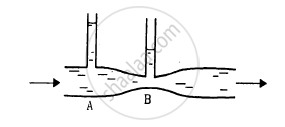Advertisement Remove all ads

# Water Flows Through a Horizontal Tube as Shown in Figure. - Physics

Sum

Water flows through a horizontal tube as shown in figure. If the difference of heights of water column in the vertical tubes is 2 cm, and the areas of cross section at A and B are 4 cm2 and 2 cm2 respectively, find the rate of flow of water across any section.Advertisement Remove all ads

#### Solution

Given:
Difference in the heights of water columns in vertical tubes = 2 cm
Area of cross section at A, aA = 4 cm2
Area of cross section at B, aB = 2 cm2
Now, let vA and vB be the speeds of water at A and B, respectively.
From the equation of continuity, we have:

$v_A a_A = v_B \times a_B$

$\Rightarrow v_A \times 4 = v_B \times 2$

$\Rightarrow v_B = 2 v_A . . . (i)$

From Bernoulli's equation, we have:

$\frac{1}{2}\rho v_A^2 + ρg h_A + p_A = \frac{1}{2}\rho v_B^2 +ρg h_B + p_B$

$\Rightarrow \frac{1}{2}\rho v_A^2 + p_A = \frac{1}{2}\rho v_B^2 + p_B$

$\Rightarrow p_A - p_B = \frac{1}{2}\rho\left( v_B^2 - v_A^2 \right)$

Here,
pA and pB are the pressures at A and B, respectively.
hA and hB are the heights of water columns at point A and B, respectively.
ρ is the density of the liquid.
Thus, we have:

$\frac{1}{2} \times 1 \times \left( 4 v_A^2 - v_A^2 \right)$

$\Rightarrow 2 \times 1 \times 1000 = \frac{1}{2} \times 1 \times 3 v_A^2$

$[ p_A - p_B = 2 cm = 2 \times 1 \times 1000 {\text{ dyne }/ {\text{cm}}^2} (\text{water column})]$

$\Rightarrow v_A^2 = \sqrt{\frac{4000}{3}} = 36 . 51 cm/s$

$\therefore\text{ Rate of flow }= v_A a_A = 36 . 51 \times 4$

$= 146 {\text{ cm}}^3 /s$

Hence, the required rate of flow of water across any section is 146 cm3/s.

Concept: Equation of Continuity
Is there an error in this question or solution?
Advertisement Remove all ads

#### APPEARS IN

HC Verma Class 11, 12 Concepts of Physics 1
Chapter 13 Fluid Mechanics
Q 32 | Page 275
Advertisement Remove all ads
Advertisement Remove all ads
Share
Notifications

View all notifications

Forgot password?